Integers 6
(10 Questions)
CA Math Standards
Grade 5
2.1 Add, subtract, multiply and divide with decimals; add with negative numbers; subtract positive integers from negative integers; verify the reasonableness of the results.
From Mr. Anker Tests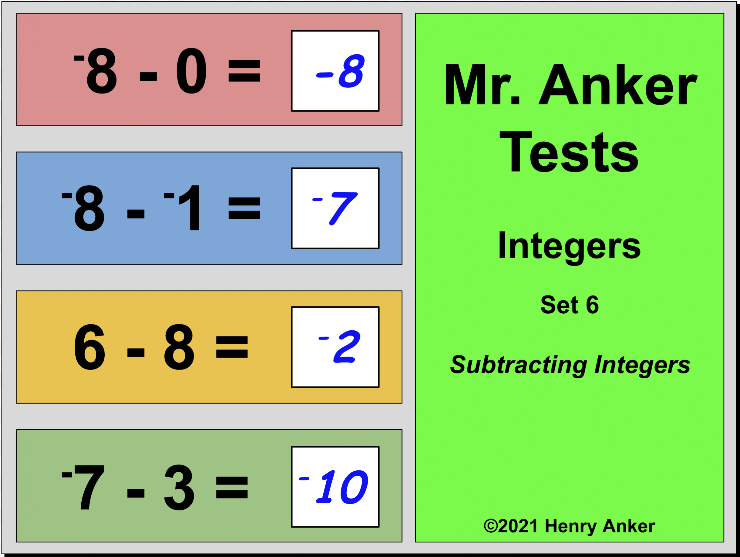Integer Number Line Reference1. Which of the choices below is the correct solution to this equation? *
1 point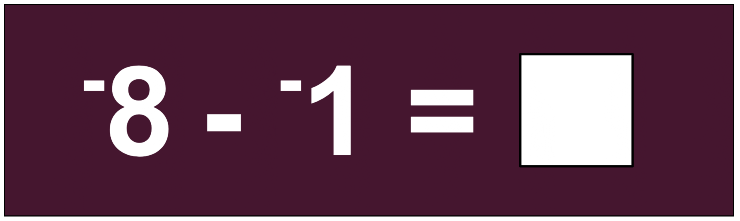*Hint: A good strategy to use is to "add the opposite". Think [-8 + 1] =
2. In the space provided below, type the correct solution to this equation. *
1 point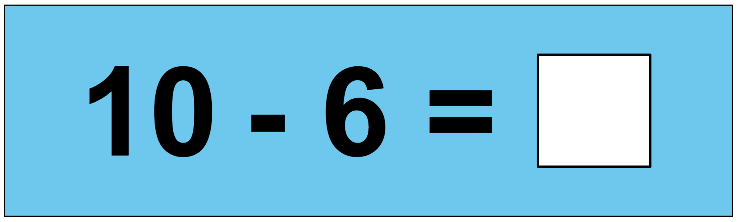3. Which of the choices below is the correct solution to this equation? *
1 point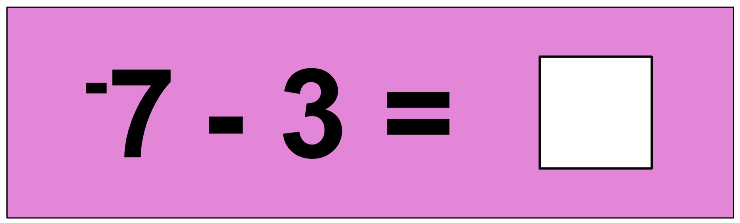4. In the space provided below, type the correct solution to this equation. *
1 point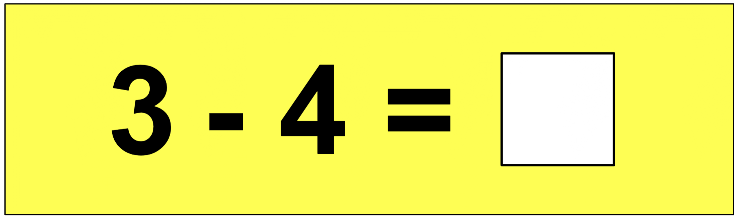5. Which of the choices below is the correct solution to this equation? *
1 point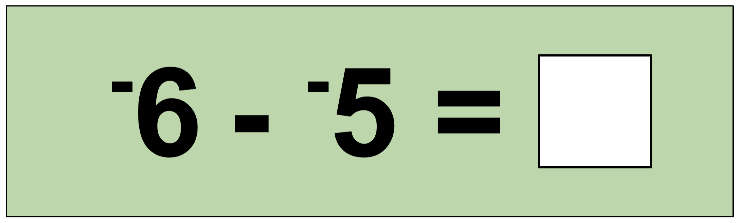6. In the space provided below, type the correct solution to this equation. *
1 point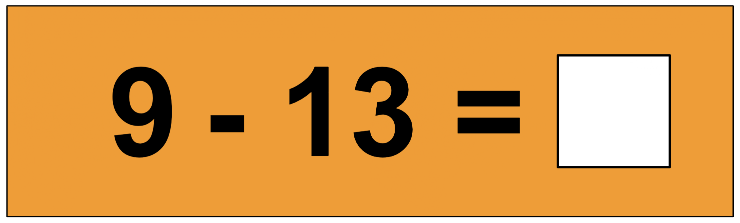7. In the space provided below, type the correct solution to this equation. *
1 point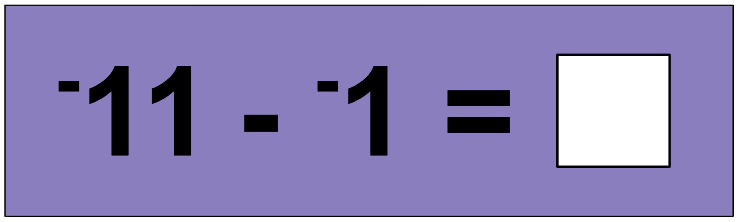8. In the space provided below, type the correct solution to this equation. *
1 point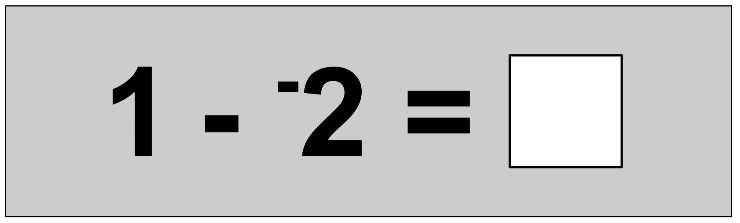9. In the space provided below, type the correct solution to this equation. *
1 point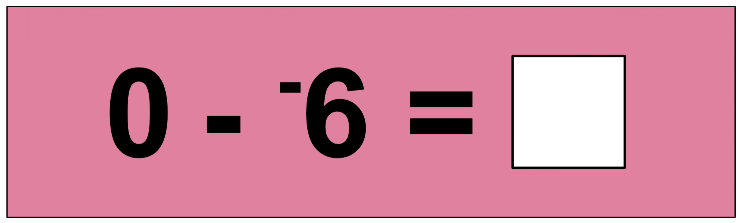10. In the space provided below, type the correct solution to this equation. *
1 point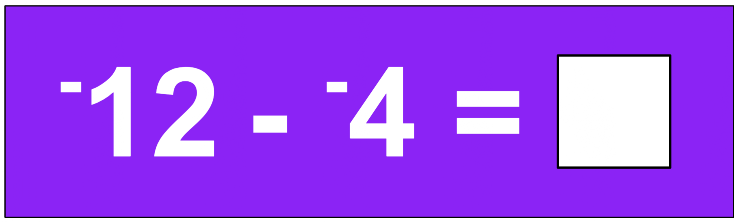Never submit passwords through Google Forms.
This content is neither created nor endorsed by Google. Report Abuse - Terms of Service - Privacy Policy